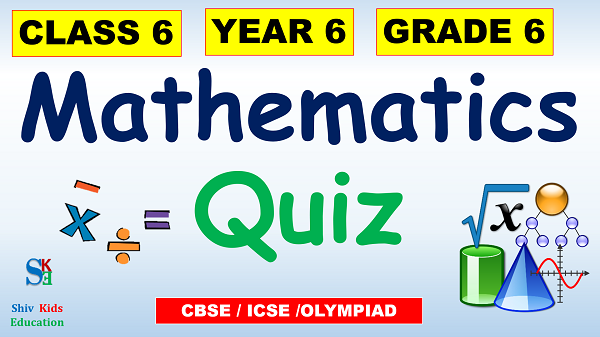# Maths quiz for class 6

## Maths quiz for class 6### Maths quiz for class 6

Mathematics, most boring subject of our school time. Let’s make it interesting and test our knowledge with Mathematics Question Answers for class 6 students. The quiz is in format of multiple-choice question (MCQ) format. It includes 25 questions which are based on unique Mathematics Quiz which enhance knowledge of Grade 6 kids. We also include many references like NCERT which will help Year 6 kids to improve their knowledge. Also include other courses like ICSE which improve skill of Grade 6 students who are very keen to learn something new.

Class 6 students who are interested in Olympiad quiz will also get great help from this Mathematics Quiz . Now let’s begin our journey to learn something new with great fun from Mathematics Quiz for Class/Grade 6 students with Shiv Kids Education.

In this website, you are going to practice for Mathematics MCQ for Class 6 students in very easy way. You will get 25 questions with multiple choice. If you would like to check your answer is correct or not then click on Show Answer button. At the end of practice, you will get result out of 25 quizzes.

You can also get class 6 GK quiz, Class 6 science quiz and grade 6 english quiz on our website SHIV KIDS EDUCATION. In addition, there are so many subject wise quizzes are available for different classes. Regularly, We would add more quiz for Year 6 Maths exercise so your children can do more practice about it. If you wish to do a practice of Mathematics through video ,Please click on Grade 6 mathematics Test

### Maths trivia questions for 6th graders

1) What fraction of an hour is 15 minutes?
1/2
1/4
3/4
2) The longest cord of a circle is called _____________.
Area
Diameter
3) What is the answer to the equation below? (‘/’ is indicates “Divided By”)
545 / 545 – 545 / 545 =
1
0
545
4) 3/7 is an improper fraction.
True
False
Not considerable
5) A triangle whose all sides and angles are equal is known as __________ triangle.
Square
perpendicular
Equilateral

### Maths quiz for class 6

6) What is the smallest 8 digit number?
10000000
11111111
10000001
7) How many degrees are there in a circle?
180 degree
360 degree
90 degree
8) How many months make a century?
12
1200
120
9) What is the square root of 441?
11
31
21
10) 25% of Rs 2500 is equal to ________.
625
125
1250

#### Math trivia questions for Grade 6 – More

11) An octagon is a polygon with ____ sides.
10
6
8
12) What are the three positive numbers that give the same result when multiplied and added together?
1,4,2
1,2,3
1,3,4
13) What is the value of ‘a’ from the below given equation?
3a – 5 = 7
3
5
4
14) Write the following in mathematical form using signs and symbols.
6 times of ‘p’ increased by 5 gives 23.
6 + 5p = 23
6p + 5 = 23
6p + 5p = 23
15) Find the perimeter of rectangle with length:18 and width:10
180
560
56
16) Every polygon has at least three sides.
False
True
Not considerable
17) Find the sum of the greatest and the smallest numbers formed by the digits 6,1,5,3 and 4 without repeating any digit.
87778
78887
88887
18) Find the value of the missing number in the calculation bellow:
25 x ( __ + 65) = (25 x 18) + (25 x 65).
18
10
20
19) The numbers which are not multiples of 2 are known as ________.
Even
Odd
Integers
20) Length of the boundary of a shape is known as its ___.
Area
Diameter
Perimeter
21) The simplest form of 12/24 is:
2/3
1/2
1/3
22) Which of these lies between 6.2 and 6.6?
6.3
6.06
6.1
23) The value of pi is equal to________.
11/7
22/7
24) The square of 9 is equal to_________.
64
108
81
25) If Y is the side of cube, then the volume of the cube is_________.
Y*Y
Y*Y*Y
3*Y